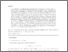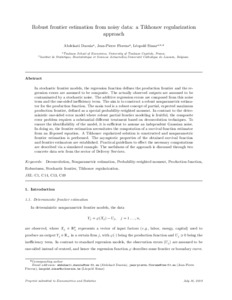# Robust frontier estimation from noisy data: a Tikhonov regularization approach

Daouia, Abdelaati, Florens, Jean-Pierre and Simar, Léopold (2016) Robust frontier estimation from noisy data: a Tikhonov regularization approach. TSE Working Paper, n. 16-665, ToulousePreview
Text
Official URL: http://tse-fr.eu/pub/30543

## Abstract

The aim of this paper is to construct a robust nonparametric estimator for the production frontier. The main tool is a concept of robust regression boundary defined as a special probability-weighted moment (PWM). We first study this problem under a regression model with one-sided errors where the regression function defines the achievable maximum output, for a given level of inputs-usage, and the regression error defines the inefficiency term. Then we consider a stochastic frontier model where the regression errors are assumed to be composite. It is more realistic to assume that the actually observed outputs are contaminated by a stochastic noise. The additive regression errors in the frontier model are then composed from this noise term and the one-sided ineficiency term. In contrast to the one-sided error model, where the direct use of empirical PWMs is fruitful, the composite error problem requires a substantial different treatment based on deconvolution techniques. To ensure the identifiability of the model we can only assume an independent Gaussian noise. In doing so, the estimation of the robust PWM frontiers, including the true regression boundary, necessitates the computation of a survival function estimator from an ill-posed equation. A Tikhonov regularized solution is constructed and nonparametric frontier estimation is performed. We unravel the asymptotic behavior of the resulting frontier estimators in both one-sided and composite error models. The procedure is very easy and fast to implement. Practical guidelines to effect the necessary computations are described via a simulated example. The usefulness of the approach is discussed through two concrete data sets from the sector of Delivery Services.

Item Type: Monograph (Working Paper) English June 2016 Toulouse Deconvolution, Nonparametric estimation, Probability-weighted moment, Production function, Robustness, Stochastic frontier, Tikhonov regularization C1 - Econometric and Statistical Methods - GeneralC13 - EstimationC14 - Semiparametric and Nonparametric MethodsC49 - Other B- ECONOMIE ET FINANCE TSE-R (Toulouse) Université Toulouse 1 Capitole UT1 04 Jul 2016 08:18 20 Nov 2018 12:35 oai:tse-fr.eu:30543 http://publications.ut-capitole.fr/id/eprint/22191

### Actions (login required)View Item